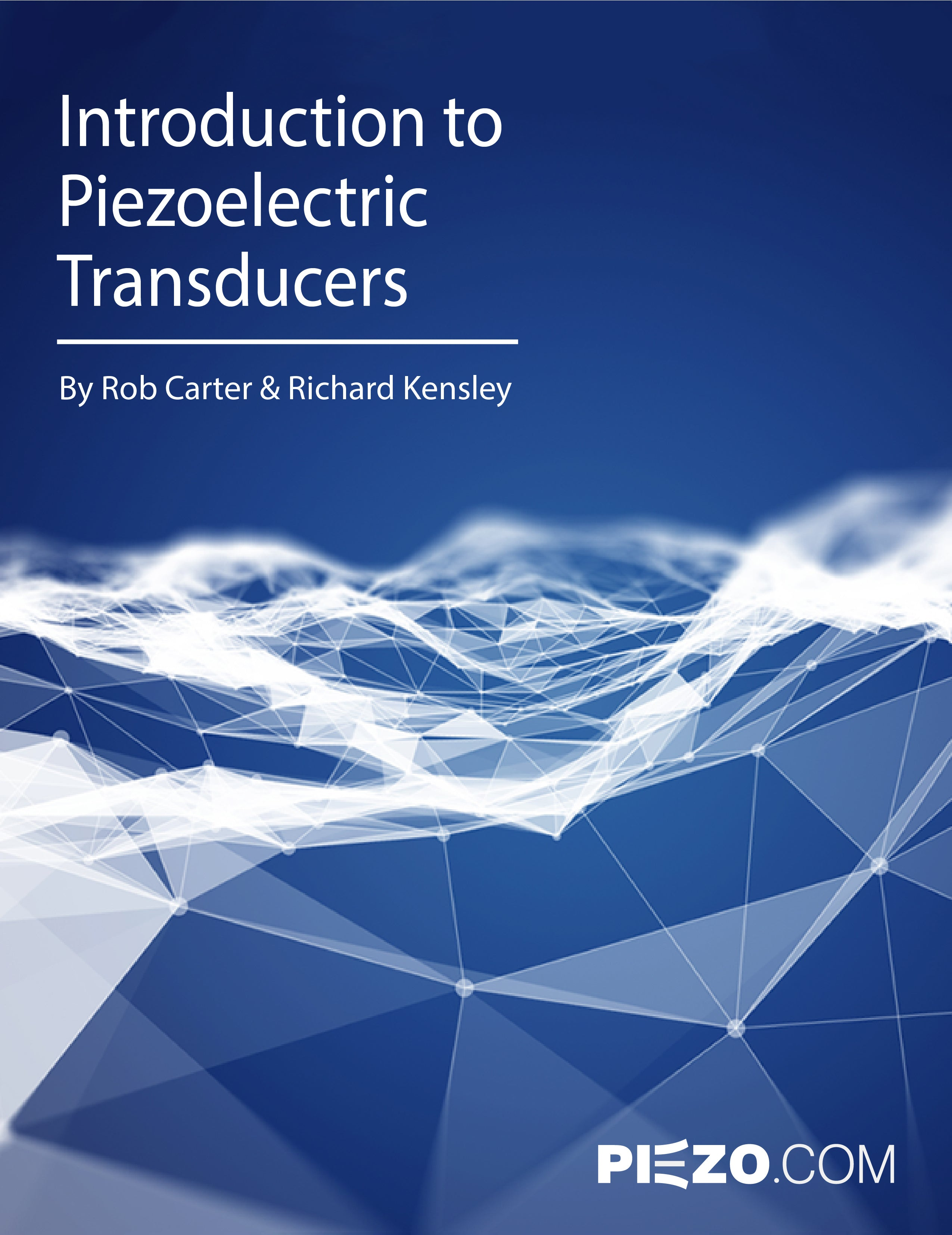Introduction to Piezoelectric Transducers
by Rob Carter and Richard Kensley
CHAPTER 1

# Introduction to Piezoelectricity

Piezoelectric Phenomenon

Piezoelectricity is a property of certain dielectric materials to physically deform in the presence of an electric field, or conversely, to produce an electrical charge when mechanically deformed. There are a wide variety of materials which exhibit this phenomenon to some degree, including natural quartz crystals, semi-crystalline polyvinylidene polymer, polycrystalline piezoceramic, bone and even wood.

Piezoelectricity is due to the spontaneous separation of charge with certain crystal structures under the right conditions. This phenomenon, referred to as spontaneous polarization, is caused by a displacement of the electron clouds relative to their individual atomic centers, i.e., a displacement of the positive ions relative to the negative ions within their crystal cells. Such a situation produces an electric dipole.

Polycrystalline ceramic, one of the most active piezoelectric materials known, is composed of randomly oriented minute crystallites. Each crystallite is further divided into tiny “domains,” or regions having similar dipole arrangements. The overall effect of randomly oriented polar domains is an initial lack of piezoelectric behavior. However, the material may be induced to exhibit macroscopic polarization in any given direction by subjecting it to a strong electric field, as shown in Figure 1. Such inducible materials are termed ferroelectric. Polarization is accomplished by applying a field of ~2350 volts/mm (60 V/mil) across electrodes deposited on outer surfaces. Once polarized, the ferroelectric material will remain polarized until it is depoled by an opposite field or elevated temperature.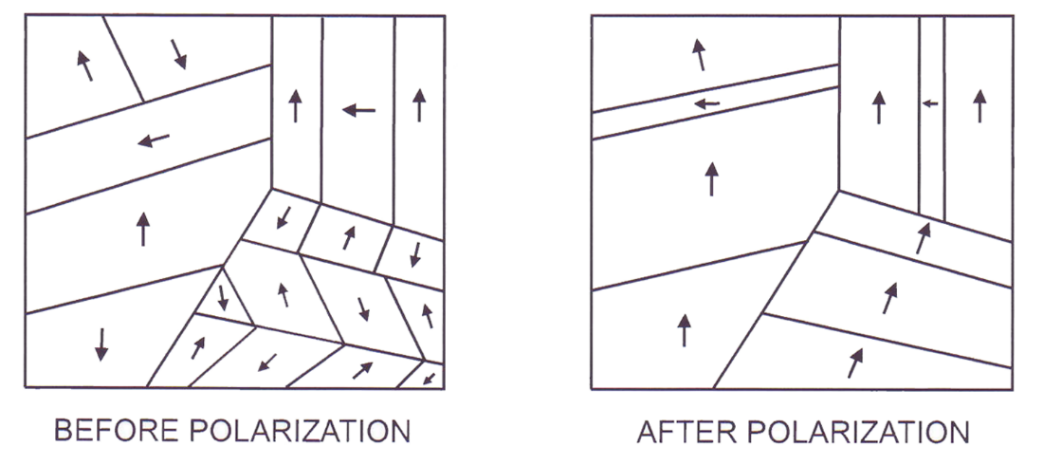Figure 1.1. Inducing macroscopic polarization in a polycrystalline piezoceramic by applying a strong electric field across randomly oriented microscopic polar domains

During electrical polarization, the material elongates permanently in the direction of the poling field (polar axis) and contracts in the transverse direction. When voltage is subsequently applied to the poled material in the same direction as the poling voltage, the piece experiences further elongation along the polar axis and transverse contraction as stipulated by Poisson’s ratio. When the voltage is removed, the piece reverts to its original pole dimensions. When voltage is applied opposite to the poled direction (depoling direction), the piece contracts along the polar axis and expands in the transverse direction. Again, it reverts to its original poled dimensions after removing the voltage. These distortions are illustrated in Figure 2 for a rectangularly shaped piece. If too large a voltage is applied in the depoling direction, the original polarization will be degraded (partially or fully depolarized). Or, the electric dipoles may be partially or completely flipped around 180°, causing the piece to be repoled in the opposite direction. The maximum depoling field a piece can withstand without experiencing depolarization is its coercive field, Ec.

When stress is applied along the poling axis, an electric field arises within the body which tends to oppose the force acting upon it. Compressive stress generates an electric field with the same orientation as the original poling field, trying to induce the piece to elongate in opposition to the compressive forces. The piece reverts to its original poled dimensions after removing the stress. Tensile stress generates an electric field with an orientation opposite to that of the original poling field. These electric fields are illustrated in Figure 2 for a rectangularly shaped piece.Figure 1.2. Physical deformation of a rectangular piezoelectric body under the influence of an applied electric field

CHAPTER 2
Piezoelectric and Material Properties of Piezoceramic
TERMINOLOGY AND RELATIONS

This section describes the terminology commonly used in the discussion of piezoceramics and notes the fundamental relationships useful in motor applications. It also defines commonly used notations and sign conventions.

Relationships between applied electric fields and the resultant responses depend upon the piezoelectric properties of the ceramic, the geometry of the piece, and the direction of electrical excitation. The properties of piezoceramic vary as a function of both strain and temperature. It should be recognized that the data commonly presented represents values measured at very low levels at ~20°C.

Directions are identified using the three axes, labeled 1, 2 and 3, shown in Figure 3. The “polar” or 3-axis is chosen parallel to the direction of polarization.

In order to conveniently express all the relevant terms, 2nd order tensors such as stress and strain are expressed as 6x1 matrices with only the unique terms listed, as demonstrated by the stress terms in Eq. (1). This allows 3rd and 4th order tensors to be expressed as 6x3 and 6x6 matrices.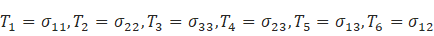(2.1)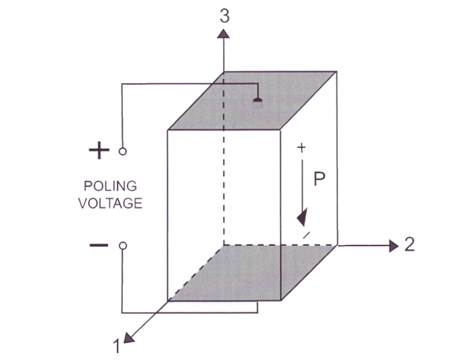Figure 2.1. Definition of axes for a rectangular piezoelectric body showing the polar or 3-axis, and the transverse or 1 and 2-axis

The polarization vector, established during manufacture by a high DC voltage applied to the electrodes, is represented by an arrow pointing from the positive to the negative poling electrode. This information is conveyed by a dot or stripe on the electrode surface held at high voltage during the poling process.

It is helpful to remember that the polarization arrow represents the force exerted on a positive charge by a positive potential field. Thus, it signifies the direction a positively charged particle would displace when influenced by a nearby positive charge. “Conventional” (positive) current flow moves in this direction. It should be kept in mind that electron flow moves in the opposite direction.

Piezoelectric coefficients, relating input parameters to output parameters, use double subscripts. The first subscript denotes the direction of the electric field E or dielectric displacement D, and the second subscript refers to the direction of mechanical stress T or strain S.

The piezoelectric charge coefficient is a 3rd order tensor that can be expressed as a 3x6 matrix that correlates the charge displaced unit area (with electrodes short circuited), associated with an applied stress, according to the relation: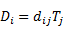(2.2)

where dij is the piezoelectric charge coefficient [Coulombs/Newton], D is the dielectric displacement [C/m2], and T is the applied stress [N/m2]. The first subscript gives the direction of the charge motion associated with the applied stress. The second subscript gives the direction of mechanical stress. D33 relates the ratio of charge motion along the 3-axis to the stress applied along the 3-axis, assuming the electrodes are shorted, and no other stresses are present. D31 relates the charge flow along the 3-axis to the stress along the 1-axis (or 2-axis) under similar conditions.

The piezoelectric voltage coefficient, g, is a 3 x 6 matrix and correlate the electric field, E, developed (with electrodes open circuited), associated with an applied stress, T, according to the relation: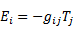(2.3)

Tensile stress is positive, compressive stress is negative, and gij is expressed in units of volts / meter per Newton / meter2. The first subscript gives the direction of the electric field associated with the applied stress. The second subscript gives the direction of mechanical stress. g33 relates the ratio of the electric field developed along the 3-axis to the stress applied along the 3-axis, assuming the electrodes are open circuited, and no other stresses are present. g31 relates the ratio of the electric field along the 3-axis to the stress along the 1-axis (or 2-axis) under similar conditions.

Piezoelectric coefficients, used to relate input parameters to output parameters, use double subscripts. The piezoelectric strain coefficients, dij, correlate the strain produced by an applied electric field according to the relation:(2.4)

Where dij is expressed in meters/volt. The first subscript gives the direction of the electric field associated with the applied voltage. The second subscript gives the direction of mechanical strain. d33 relates the ratio of the strain along the 3-axis to the electric field applied along the 3-axis, assuming the piece is free to distort in any direction. d31 relates the strain along the 1-axis (or 2-axis) to the excitation along the 3-axis under similar conditions.

The coupling coefficient, k (lower case), is an indication of the materials ability to convert electrical energy to mechanical energy. Specifically, the square of the coupling coefficient equals the ratio of mechanical energy output to the electrical energy input. It is more relevant to the maximum work output of solid ceramic devices than to bending elements because a practical bending element stores a portion of its mechanical energy in the mount and metal shim center layer. For a bending element keffective ~ ¾ k31.

The relative dielectric constant, K (upper case), is the ratio of the permittivity of piezoceramic to that of empty space, ε0 (ε0 = 8.854x10-12 farads/meter). K3, the relative dielectric constant between the poling electrodes, determines the capacitance of the piece according to the relationship,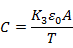(2.5)

Where A is the area of the surface electrode, and T is the thickness of the ceramic layer or layers between electrodes.

Certain piezoceramic material constants are written with superscripts to specify the experimental context in which the parameter is measured.

T = Constant Stress (mechanically free)
S = Constant Strain (mechanically clamped)
E = Constant Electric Field (electrodes short-circuited)
D = Constant Electric Displacement (electrodes open-circuited)

For example, KT3, is the dielectric constant measured across the poling electrodes of a mechanically free piece.

Young’s Modulus, Y, the ratio of stress required to produce a unit of strain, describes the material stiffness of piezoceramic in units of newtons/square meter. Because mechanical stressing of the ceramic produces an electrical response opposing the resultant strain, the effective Young’s Modulus with electrodes short circuited is lower than with the electrodes open circuited. Furthermore, the stiffness differs in the 3 direction from that of the 1 or 2 direction. This, YE11, is the ratio of stress in the 1 direction to stain in the 1 direction with the electrodes shorted.

PIEZOELECTRIC AND MATERIAL PROPERTIES FOR PZT-5A PIEZOCERAMIC

The piezoelectric and material properties of PZT-5A piezoceramic are listed in Table 1.Figure 2.2. TABLE 1. PIEZOELECTRIC AND MATERIAL PROPERTIES FOR PZT-5A CERAMIC
MATERIAL PROPERTIES FOR PIEZOCERAMICS

The piezoelectric and other material properties of PZT-5A, PZT-5H and PZT-5J piezoceramic are listed in Table 2.Figure 2.3. TABLE 2. PIEZOELECTRIC AND MATERIAL PROPERTIES FOR PZT-5H, PZT-5A, AND PZT-5J PIEZOCERAMIC